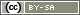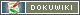# Variables in SoSci Survey

There is frequent mention of “variables” in this manual. This chapter explains how the different variables can be used.

Variables are used normally in social sciences for statistical analysis. A variable is identified here as a characteristic of the survey unit (e.g. age, gender). The variable “varies” insofar, as we know, in that there is a characteristic and that it affects the analysis – but the effect depends on the actual case.

In principle, a variable is very similar in the programming world: the variable describes a variable value which is used. This could be, for example, the participant's response – but it could also be a value that changes several times while the questionnaire page is still being developed. The variable is always used as a placeholder for variable (numerical) values, as we know from variables in mathematics. Technically, a variable is a storage place for data (e.g. for a number or text).

You will come across the following types of variables in SoSci Survey:

• PHP variables are a feature of the programming language PHP. They appear directly in the PHP code (i.e. a PHP code element when composing the questionnaire), and start with a dollar sign (`\$`), e.g. `\$age`. PHP variables are described in detail below.
• Data set variables store the participant's responses – they are accessible later on in the data record as variables for the analysis. Each questionnaire that is filled out generates a new case in the data record. A list of all data variables in a survey project can be seen in the Variables Overview. The current value of a data variable, for example, the answer given by a respondent, can be read with the function `value()` and used in a filter.
• Internal Variables are specific data variables. They are used to make values in the data record accessible that were calculated with PHP code or drawn. In addition, internal variables can save responses that were collected with custom form elements.

## Data Set Variables

Most questions create a variable in the data set, moreover you can use the question type Internal Variables (see below) to create additional variables in the data set that store user-defined data.

In addition, there are additional variables containing meta and para data for each record.

When questions are created, the variables are assigned an ID consisting of the question ID (e.g. “AB01”) and (optionally) an suffix (e.g. “_01” or “x1”). For questions with subordinate items (e.g. scales), the suffix usually corresponds to the number of the item.

### Custom Variable IDs

In the tab “Variables” of a question you can change the ID of one or more variables.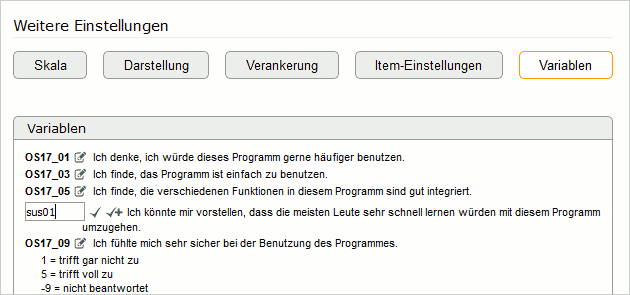Click the edit button (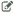) to change the ID. Enter the desired new ID for the variable. The ID must comply with the following rules:

• The letters A to Z (upper or lower case), the digits 0 to 9 and the underscore (`_`) can be used. The first character must be a letter.
• The IDs can be a maximum of 64 characters long.
• To avoid possible overlaps with the automatically assigned IDs, the third and fourth characters must not be both digits. The identifiers “var01”, “v01”, or “age” are therefore possible, but not “va00”, “v001”, or “xy123”.

If you do not enter anything in the input field for the ID, the automatic ID is restored.

Two buttons are available to set the new ID:

• The button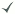sets the ID only for the one variable.
• The button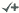additionally sets the IDs for all subsequent variables, with SoSci Survey automatically incrementing a number at the end. For example, if you enter the ID “sus01” for the first variable, the other variables are assigned the IDs “sus02, “sus03”, and so on.

The changed identifiers are used as variable names in Download Data and can be used in PHP-Filters or the function `value()`. The automatically assigned ID also remains valid.

## Internal Variables

Create a question with the type Internal Variables to insert an internal variable. Now add one or more variables to this question – just like how you add options to a selection question. The description you enter for a variable will appear later on in the data record as the label for the variable.

Note: The ID of an internal variable consists of 7 characters, e.g. “IV01_01” or “AB02_03” – if you are unsure, check in the Variables Overview. Only use the ID of the primary question (e.g. “IV01” or “AB02”) when you are actually referring to all of the internal variables in the question (e.g. with `urnDraw()`), and not just to a single variable.

Internal variables have different functions: you can use them with `put()`, in the context of a randomization with urns, or together with custom form elements.

### Use with put()

An internal variable can store a value that was drawn or generated in PHP code. The function `put()` simply writes the value to the variable.

In the following example, the sum index for the scale “SK01” is calculated and stored in the internal variable `SK02_01`. This can be useful if you are at loggerheads with your statistical software – or if you still want to use the scale index in the questionnaire for filters.

```\$sum = valueSum('SK01');
put('SK02_01', \$sum);```

Below you will find another example that makes the result of a random draw available for the analysis by using `put()`.

### Use with Urns

The use of urns can be read in detail in the chapter Randomization Using Urns.

Ballots with several values can be stored in an urn – in some circumstances, this means multiple values have to be stored in multiple internal variables. The function `urnDraw()` expects the ID of the question accordingly. In turn, this question has to contain enough variables in order to be able to store the combination drawn.

The following PHP code draws a ballot out of the urn “urn” and stores the combination in the internal variable in question “IV01”.

`urnDraw('urn', 'IV01');`

In the simplest and most common scenario in that there are only ballots with one each number each in the urn, the question only requires one internal variable. Nevertheless, the function `urnDraw()` requires the question ID, not the variable ID.

### Use with JavaScript

If a question type “internal variables” is dragged into the questionnaire, it will not be represented as a question and so nothing can be seen. However, there are two effects in doing so:

1. Hidden (`type="hidden"`) form fields with the IDs of the internal variables will be created on the questionnaire page.
2. When the page is submitted with the “Next” button, the values from the hidden form fields will be read and stored in the data record.

The hidden form fields can be accessed by using JavaScript. This means responses or measured values gathered with JavaScript can be conveniently stored in the data record.

The following example assumes that a question type “internal variables” with ID “PC01” was created with two variables in it, which have IDs “PC01_01” and “PC01_02”. The hidden form element for variable “PC01_01” can be accessed in JavaScript by using `document.getElementById("PC01_01")`. The width and height of the screen should be generated (in pixels) via JavaScript, and stored in the data record.

In addition, question “PC01” is dragged onto the questionnaire page, and the following JavaScript code is placed underneath it as a text element.

```<script type="text/javascript">
<!--
document.getElementById("PC01_01").value = screen.width;
document.getElementById("PC01_02").value = screen.height;
// -->
</script>```

## PHP Variables

In PHP, variables start with a dollar sign, e.g. `\$version`. A value is assigned to the variable by using a single equals sign (`=`). This value is compared with another value by using a double equals sign (`==`).

Note: A PHP variable is only valid within a PHP code element. It is transient, and therefore will not be stored permanently. If you want to use the variable later on in other PHP code elements, the variable therefore has to be made available by using `registerVariable()`. If the value of the variable is needed for the analysis, it has to be stored in an internal variable by using `put()`.

In the following example, the function `random()` rolls number 1 or 2. The result of this random draw is stored in the variable `\$version`. Afterwards, an IF construction (Filters and Conditional Questions) is used to compare if it is value 1 (then the text element “stimulus1” will be displayed) or not (then “stimulus2” will be displayed).

```\$version = random(1, 2);
if (\$version == 1) {
text('stimulus1');
} else{
text('stimulus2');
}```

### Save in the Data Record

In order to save a variable's content beyond the questionnaire, use an internal variable (see above), combined with the function `put()`.

In the example above, the stimulus that was displayed cannot be seen with the data record. Create an internal variable (e.g. “IV01_01”) and use `put()` in order to save the result of the random draw in the data record:

```\$version = random(1, 2);
put('IV01_01', \$version);
if (\$version == 1) {
text('stimulus1');
} else{
text('stimulus2');
}```

### Working with Variables

Variables allow you to calculate superbly. In the following example, the participant is asked question “AB01” on page 1 – a text input for a number. The response is read on page 2 using `value()` and stored in the variable `\$number`. This variable is multiplied by 2 (`*`) and the result is stored in the variable `\$result`.

Subsequently, the variable `\$result` is treated as text (string) and linked with two other string (`.`). The result of this link is transferred to the function `html()` to display in the questionnaire.

```// Calculate on page 2 with the given number
\$number = value('AB01');  // assign given number to the variable \$number
\$number = (int)\$number;   // make sure that it is a number
\$result = 2 * \$number;  // multiple number by 2
// Display as text in the questionnaire
html('<p>You have specified half of '.\$result.' </p>');```

### Use Variables Continuously

Sometimes it is necessary to use one PHP variable in multiple PHP code elements. In the following example, 10 television stations will be queried in a random order. In question AB01 the participant will be asked how often they watch the stations – question AB02 will enquire as to how appealing they find the program schedule.

It makes sense that the stations are queried in the same order in both questions. In order to do so, first of a list (Array) of the item IDs in a random order is created using `random_items()`. This list is stored in the variable `\$items`.

The variable `\$items` is not only needed on the current page, but on the following page for question “AB02” as well. This is therefore made accessible for future PHP code elements using `registerVariable()`. Subsequently, question “AB01” will be displayed in the shuffled order.

```\$items = random_items('AB01'); // randomized order of items in question AB01
registerVariable(\$items);
question('AB01', \$items);  // ask question with randomized items```

`\$items` can then simply be used on page 2.

`question('AB02', \$items);  // ask question with randomized items. `